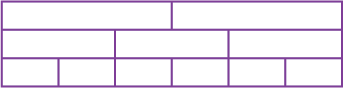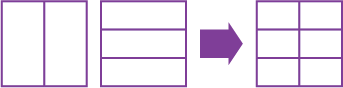Home > Fractions > Good teaching > Equivalence > Grids and arrays

# Grids and arrays

The most valuable aspect of using grids and arrays is that they focus thinking on factors and multiples, which is the essential foundation for developing general strategies for creating equivalent fractions.

Representations such as circles and fraction walls are more likely to promote additive thinking.

For example, this fraction wall clearly shows that $$\frac{3}{6}$$ is equivalent to $$\frac{1}{2}$$ but tends to suggest the additive relationship of $$\frac{1}{6}$$ + $$\frac{1}{6}$$ + $$\frac{1}{6}$$ = $$\frac{1}{2}$$.A fraction wall.

On the other hand, having to create the one grid that will show both halves and sixths demands thinking about the multiplicative relationship between the denominators 2 and 6 (i.e. 2 $$\times$$ 3 = 6).Combining grids.

The aim of activities with grids and arrays is to develop a conceptual understanding of the processes for recognising and creating equivalent fractions.

Students need time to explore these processes with models rather than simply learning an abstract procedure for forming equivalent fractions.

## Overlay grids

Pre-prepared squares, divided into equal parts using vertical (or horizontal) lines, are overlayed to create grids depicting smaller parts. This helps students to work with factors and multiples to find equivalent fractions.

## Dynamic fractions

Two numbers are entered into a digital tool to construct a grid. Boxes on the grid are then shaded to represent fractions, and equivalent fractions determined.